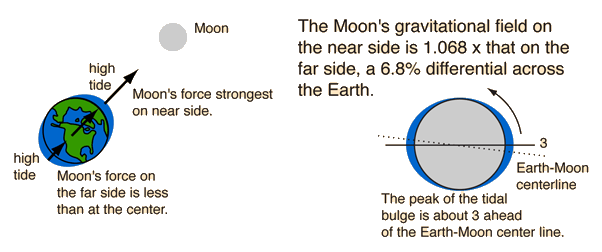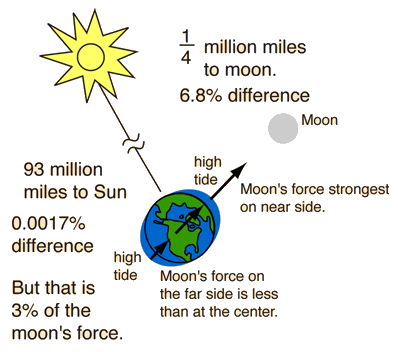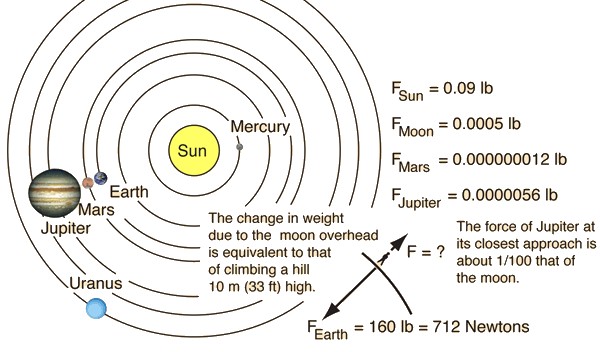# Tides

The Earth experiences two high tides per day because of the difference in the Moon's gravitational field at the Earth's surface and at its center. You could say that there is a high tide on the side nearest the Moon because the Moon pulls the water away from the Earth, and a high tide on the opposite side because the Moon pulls the Earth away from the water on the far side. The tidal effects are greatly exaggerated in the sketches.The tidal influence on a close object is greater because the inverse square law drop in gravitational force gives a greater ratio of the force on the near side of the object to that on the far side. As shown below, the tidal ratio of the force per unit mass on the near side compared to that on the far side is much larger for the closer object.Why is the moon's tidal effect greater than the Sun's?
How large is the Sun's tidal effect compared to the moon's influence?
Index

Gravity concepts

 HyperPhysics***** Mechanics R Nave
Go Back

# Sun's Tidal Effect

Even though the Sun is 391 times as far away from the Earth as the Moon, its force on the Earth is about 175 times as large. Yet its tidal effect is smaller than that of the Moon because tides are caused by the difference in gravity field across the Earth. The Earth's diameter is such a small fraction of the Sun-Earth distance that the gravity field changes by only a factor of 1.00017 across the Earth. The actual force differential across the Earth is 0.00017 x 174.5 = 0.03 times the Moon's force, compared to 0.068 difference across the Earth for the Moon's force. The actual tidal influence then is 44% of that of the Moon.### Why is the moon the dominant tidal influence?

Index

Gravity concepts

 HyperPhysics***** Mechanics R Nave
Go Back

# Moon as Dominant Tidal SourceThe Moon is the dominant tidal influence because the fractional difference in its force across the Earth is greater than the fractional difference seen from the Sun. This difference in force follows the inverse square law.

### Sun's tidal influence

Index

Gravity concepts

 HyperPhysics***** Mechanics R Nave
Go Back

# Jupiter Effect

A popular book warned of disastrous gravitational effects from the special alignment of the planets Jupiter and Mars along with the Moon. The forces of attraction compared to that of the Earth are illustrated for a person weighing 160 lb on the Earth's surface.Don't lose much sleep worrying about the Jupiter effect. You change the gravity force on yourself by taking one step up a stairway more than the combined gravitational effects of both Jupiter and Mars if they were perfectly aligned!

Index

Gravity concepts

 HyperPhysics***** Mechanics R Nave
Go Back